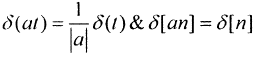Courses

# Signals & Systems - MCQ Test - 1

## 15 Questions MCQ Test GATE ECE (Electronics) 2022 Mock Test Series | Signals & Systems - MCQ Test - 1

Description
This mock test of Signals & Systems - MCQ Test - 1 for Electronics and Communication Engineering (ECE) helps you for every Electronics and Communication Engineering (ECE) entrance exam. This contains 15 Multiple Choice Questions for Electronics and Communication Engineering (ECE) Signals & Systems - MCQ Test - 1 (mcq) to study with solutions a complete question bank. The solved questions answers in this Signals & Systems - MCQ Test - 1 quiz give you a good mix of easy questions and tough questions. Electronics and Communication Engineering (ECE) students definitely take this Signals & Systems - MCQ Test - 1 exercise for a better result in the exam. You can find other Signals & Systems - MCQ Test - 1 extra questions, long questions & short questions for Electronics and Communication Engineering (ECE) on EduRev as well by searching above.
QUESTION: 1

### If a real function f(t) has Fourier transform F(ω), then Fourier transform of f(t) - f(-t) will be

Solution: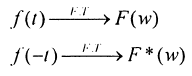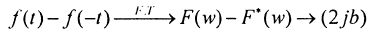If F(w)is a complex no.a+jb

then Ft (w) is (a-jb)

SoF.T.of f(t)-f(-t) will bean imaginary number equal to (2 jb)

QUESTION: 2

### If impulse response of a discrete time LTI system is an u[n] then its step response is

Solution: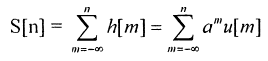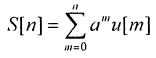QUESTION: 3

### Q. If transfer function H(s) of a system is given as H(s) =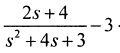< Re {s} < -1 then value of h(t) is given as.

Solution:

Take partial fraction and then take inverse according to range of ROC.

QUESTION: 4

if u(t) is unit step and δ(t) is unit impulse function then Inverse z, Transform of F(z) = 1/z + 1For k > 0 is

Solution:
QUESTION: 5

What is the value of Fundamental period of sequence x[n] = 3 sin (1.3 nπ+ π/2) + sin (1.2π)

Solution:
QUESTION: 6

If Fourier transform of x[n] is X(e'°') then Fourier transform of (- 1)n x[n] will be

Solution: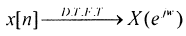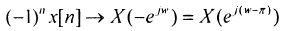QUESTION: 7

A continuous time system is described by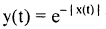where y(t) is output and x(t) is input, then y(t)is bounded.

Solution: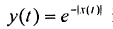if x (t) is not bounded then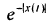is bounded.

QUESTION: 8

Magnitude of Fourier transform x(t) & y(t) are shown in figure. If phases of X(ω) and Y(ω) are zero for all w. The magnitude and frequency units are identical in both figure then relation between y(t) and x(t) is expressed as :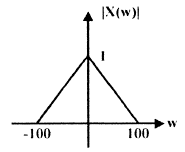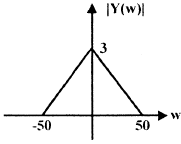Solution:
QUESTION: 9

X (z) =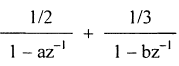, if lal & Ibl < 1 with ROC, specified as

|a| < |z| < |b| then x  of corresponding sequence is given by.

Solution: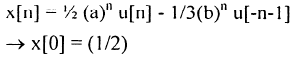QUESTION: 10

If impulse response of a system is h(t) = e-|t|then.

Solution: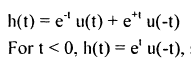so it is a non - causal system.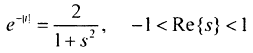So         it is a stable - system.

QUESTION: 11

A box has 4 white and 3 red balls. Two balls are taken out in succession. What is the probability that both are white?

Solution:
QUESTION: 12

For a given discrete time sequencex[n] = 2, 0, - 1, - 3, 4, 1, - 1 ) then value of X (e) is

Solution:
QUESTION: 13

Which of the following transfer function corresponds to an unstable system

Solution:
QUESTION: 14

14 If average power and energy of signal u(t) are P and E then

Solution:

Just use formula for energy and power.

QUESTION: 15

The value of δ(at) and δ(an) are respectively.

Solution: